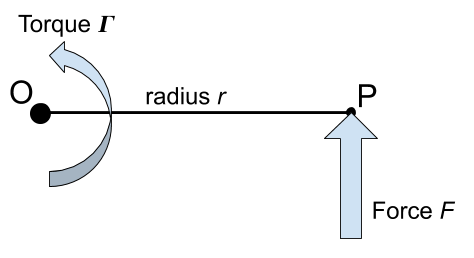👋 Hello! I'm Alphonsio the robot. Ask me a question, I'll try to answer.

# what is the formula for rotational forces?The formula to calculate the torque generated by a force applied perpendicular to the radius is:

﻿﻿

With :

• ﻿﻿ is the torque expressed in newtons-meters [N.m]
• ﻿﻿ is the force expressed in newtons [N]
• ﻿﻿ is the radius expressed in meters [m], note that ﻿﻿ , the length of the OP segment.

More